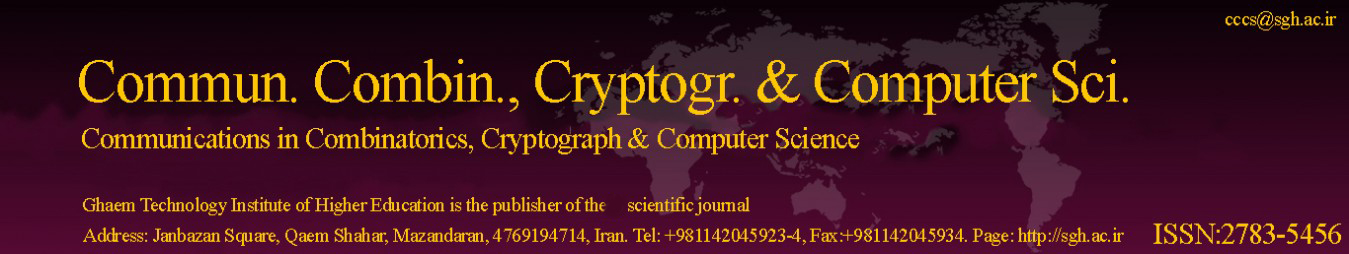Original Research Article

Article volume = 2023 and issue = 1

Pages: 61–74

Article publication Date: March 4, 2023

# Anti fuzzy B-subalgebras under S-norms

#### Rasul Rasuli

Department of Mathematics, Payame Noor University (PNU), P. O. Box 19395-3697, Tehran, Iran.

##### Abstract:

In this paper, we apply the concept of s-norm S to fuzzy structure of B-algebras. The notion of an anti fuzzy B-subalgebra and an anti fuzzy normal B-subalgebra with respect to s-norm are introduced and several related properties are investigated. The union and direct sum of them are defined and investigated. Finally, by using B-homomorphisms of B-algebras, characterizations of them are given.

##### Keywords:

Algebra and orders, theory of fuzzy sets, norms, sums and unions, homomorphisms.

##### References:
•  M. T. Abu Osman, On some products of fuzzy subgroups, Fuzzy Sets and Systems, 24(1987), 79-86. 2.14
•  S. S. Ahn and K. Bang, On fuzzy subalgebras in B-algebras, Communications of the Korean Mathematical Society, 18(3)(2003), 429-437. 1
•  P. J. Allen, J. Neggers and H. S. Kim, B-algebras and groups, Scientiae Mathematicae Japonicae Online, 9(20030), 159-165. 2.5
•  J. J. Buckley and E. Eslami, An introduction to fuzzy logic and fuzzy sets, Springer-Verlag Berlin Heidelberg GmbH, (2002). 2.6, 2.8, 2.9
•  Y. B. Jun, E. H. Roh and H. S. Kim, On fuzzy B-algebras, Czech. Math. J., 52(2002), 375-384. 1, 2.1, 2.2, 2.3, 2.4
•  D. S. Malik and J. N. Mordeson, Fuzzy Commutative Algebra, World Science publishing Co.Pte.Ltd., (1995). 2.10, 2.11, 2.12
•  J. Neggers and H. S. Kim, On B-algebras, Mate. Vesnik, 54(2002), 21-29. 1
•  J. Neggers and H. S. Kim, A fundamental theorem of B-homomorphism for B-algebras, Intern. Math. J., 2(2002), 207-214. 1
•  R. Rasuli, M. M. Moatamedi nezhad and H. Naraghi, S-norms over anti fuzzy implicative ideals, anti fuzzy positive imoplicative ideals in BCK-Algebras, The Third National Congress on Mathematics and Statistics Conbad Kavous University, Iran, Conbad Kavous, 2021. 1, 2.7, 2.13
•  R. Rasuli, T-fuzzy subbigroups and normal T-fuzzy subbigroups of bigroups, J. of Ramannujan Society of Mathematics and Mathematical Sciences, 9(2)(2022), 165-184. 1
•  R. Rasuli, M. A. Hashemi and B. Taherkhani, S-norms and Anti fuzzy ideals of BCI-algebras, 10th National Mathematics Conference of the Payame Noor University, Shiraz, May, 2022. 1
•  R. Rasuli, S - (M,N)-fuzzy subgroups, 1th National Conference on Applied Reserches in Basic Sciences( Mathematics, Chemistry and Physics) held by University of Ayatolla Boroujerdi, Iran, during May 26-27, 2022. 1
•  R. Rasuli, Intuitionistic fuzzy BCI-algebras (implicative ideals, closed implicative ideals, commutative ideals) under norms, Mathematical Analysis and its Contemporary Applications, 4(3)(2022), 17-34. 1
•  R. Rasuli, Fuzzy d-algebras under t-norms, Eng. Appl. Sci. Lett. (EASL), 5(1)(2022), 27-36. 1
•  R. Rasuli, T-fuzzy G-submodules, Scientia Magna, 17(1)(2022), 107-118. 1
•  R. Rasuli, Norms Over Intuitionistic Fuzzy Subgroups on Direct Product of Groups, Commun. Combin., Cryptogr. & Computer Sci., 1(2023), 39-54. 1
•  A. B. Saeid, Fuzzy Topological B-algebras, International Journal of Fuzzy Systems, 8(3)(2006), 160-164. 1
•  T. Senapati, M. Bhowmik and M. Pal , Fuzzy B-subalgebras of B-algebra with Respect to t-norm, Journal of Fuzzy Set Valued Anakysis, 11(2012), 1-11. 1
•  L. A. Zadeh, Fuzzy sets, Information and Control, 8(3)(1965), 338-353. 1